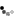# Data Assimilation Demo

This web-page aims to demonstrate the Kalman Filter with some simple linear toy models. First choose model and data assimilation parameters and then click on "Run assimilation".

Model parameters
 Model Equation(s) Model code The function model should return the state vector at the next time step given the state vector x at the time step n. function model(n,x) { // swap elements return [x,x]; } State vector size Number of time steps True initial condition xi
Data assimilation parameters
 Method (Extended) Kalman Filter Ensemble Kalman Filter Optimal Interpolation Nudging 4DVar Covariance matrix of initial condition error Pi Covariance matrix of model error Q Observed every x grid points Model time steps between observations Observation error variance Seed for random numbers Relaxation time-scale for nudging Number of ensemble members Max. number of inner loops Variance inflation Max. number of outer loopsState vector
Show: Truth Free run Assimilation Observations
Error covariance
Show: Free run Assimilation